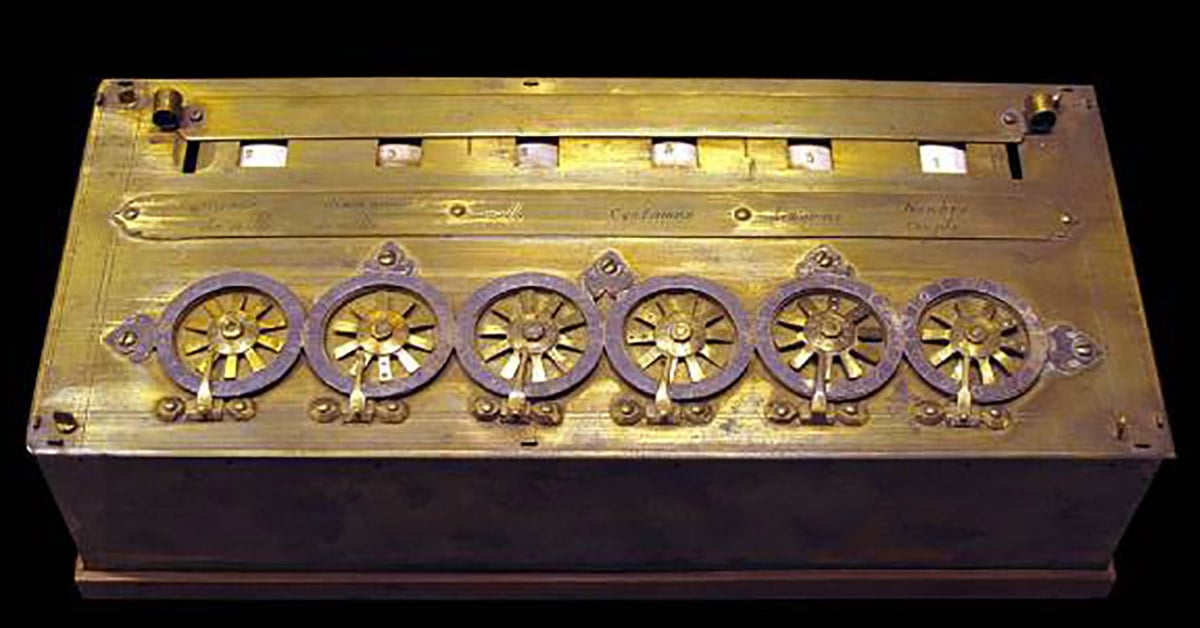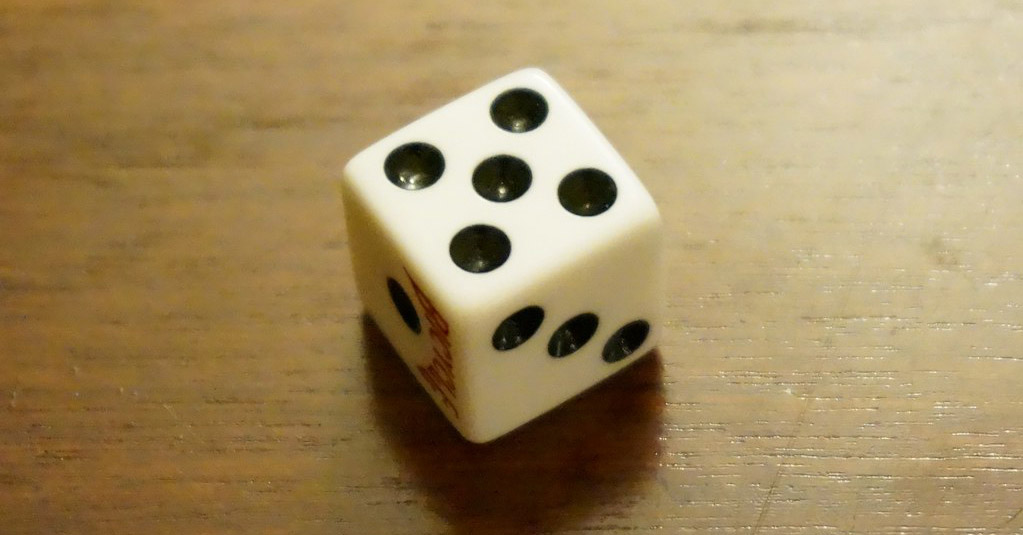# 5 Amazing Innovations and Discoveries of Blaise Pascal

Blaise Pascal, the 17th-century mathematician, inventor, and theologian was the very definition of a polymath as these incredible inventions and discoveries demonstrate.
•Few figures in mathematics, science, or philosophy enjoy as high a reputation as Blaise Pascal, but the list of Blaise Pascal’s inventions and contributions to an incredible diversity of disciplines more than justifies the praise he has received, even in his own time. Pascal’s legacy extends to everything from mechanical calculators to the hydraulic press, and much of his handiwork is either still in practical use today or provided the basis for further advancements in the centuries since.

## Pascal’s theorem

Not everyone can impress one of the greatest minds in mathematics to the point of envy before they turn 17, but Blaise Pascal did exactly that. No less than René Descartes is said to have been jealous of the young Pascal after he published an essay on conic sections entitled, “Essai pour les coniques in 1640, based on his study of Girard Desargues’s work on synthetic projective geometry.

RELATED: THE PEOPLE'S BEST FRIEND: THE CALCULATORS' BRIEF HISTORY

Pascal discovered that if any six arbitrary points on a conic projection—produced by the intersection of a plane with a cone—are joined by arbitrary line segments so they form a hexagon, then the three pairs of opposing sides of that hexagon will meet at three points along a straight line [PDF].

## The PascalineBlaise Pascal's Pascaline, the first mechanical digital calculator.      Source: Rama/Wikimedia Commons

Shortly after producing what has become known as Pascal’s theorem, Pascal went to work on another mathematical problem, but this one was a much more practical one: how to automate the adding together of integers in a mechanical device.

Managing large tables of numbers was a difficult task, and mathematicians had long relied on physical tools to assist them in keeping track of calculations, such as the Chinese or Roman abacus. Pascal’s father, Étienne, was appointed in 1639 as a local tax administrator in Rouen, France. Pascal senior didn’t need to work in advanced mathematics, but he did need a quick and easy way to perform basic computations like adding and multiplying.

To help solve this challenge, Pascal created what he modestly called the Pascaline, a mechanical integer calculator that is considered by some to be the first working digital calculator ever produced, since it operated by counting integers.

## The theory of probabilityPascal was the first to quantify the equality of probability in an honest die role.    Source: Glenn Fleishmann/Flickr

Pascal devised his theory of probability through correspondence with the renown mathematician Pierre de Fermat. It was based on a classical problem in probability theory, called the division of the stakes, which mathematicians had been discussing for around 200 years. It was one of the famous problems that motivated the beginnings of modern probability theory in the 17th century and it led Blaise Pascal to the first theories about what today is known as an expected value.

Most Popular

The problem involves a game of chance with two players. In each round, the players have an equal chance of winning. The players contribute equally to a prize pot. They also agree that the first player to win a certain number of rounds will collect the entire prize. In the problem, we suppose that the game is interrupted before either player won the stipulated number of rounds. So, the question becomes, how can the pot be divided fairly? It is assumed that a fair division would depend on the player who has won more rounds getting a larger share of the pot. But the problem is not only one of calculation; it also involves deciding what constitutes a "fair" division.

In 1654, mathematician Chevalier de Méré posed the problem to Blaise Pascal, who discussed it in correspondence with Fermat. Pascal and Fermat eventually provided a solution to this problem, and developed concepts that are still fundamental to probability theory.

The pair reasoned that the division should depend not on the number of rounds each player had won when the game was interrupted, but on the possible ways the game might have continued. In other words, what is important is the number of rounds each player needs to win in order to achieve victory. They then developed mathematical theorems to determine the possible outcomes. Pascal's analysis was an early example of using expectation values instead of odds when reasoning about probability.

## Atmospheric pressure

The substance of the atmosphere has been one of the oldest subjects of scrutiny by natural scientists and philosophers the world over. The Greeks identified air as one of their five fundamental elements of matter, but it wouldn’t be until the 17th century that the emergence of modern scientific techniques that the air around us would begin to be scientifically described.

Pascal studied the works of Galileo and the Italian physicist Evangelista Torricelli and began conducting his own experiments to measure the atmosphere. His experiments measured the atmospheric pressure and paved the way for further studies in hydrodynamics and hydrostatics. A unit of atmospheric pressure is called a Pascal in honor of this contribution.

## Pascal’s law

In his experiments on atmospheric pressure, Pascal developed several major innovations as a way to study and measure the atmosphere. One of the most widely used is the hydraulic press, which distributes force through a liquid from one place to another. This system is essential to industrial processes and machinery today and relies on what’s become known as Pascal’s law.

Pascal’s law states that “when there is an increase in pressure at any point in a confined fluid, there is an equal increase at every other point in the container.” This law and the experiments that Pascal developed to identify it were essential for the development of the modern field of hydrodynamics.SHOW COMMENT (1)